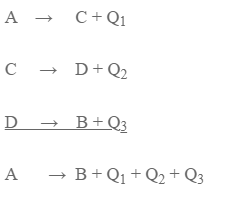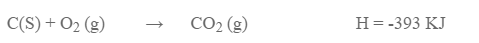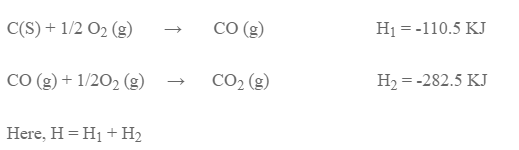# Hess’s Law Of Constant Heat Summation

Hess’s law of constant heat summation states, “The total amount of heat involved in a physic-chemical process is same whether the process is done in single step or multiple steps involving intermediates.”

A can be converted to B into two ways:

Method I: A I directly converted to B

A = B + Q

Method II: A is first converted to C, then to D and finally to BAccording to Hess’s Law,

Q = Q1 + Q2 + Q3

This law can be verified by taking the conversion of carbon to carbon dioxide.

Method I: Carbon is directly oxidized to carbon dioxide.Method II: Carbon is first converted to carbon monoxide and then to carbon dioxide.This result verifies Hess’s law of constant heat summation.

Application of Hess’s law:

This law can be used to determine heat of transition of allotropes.
This law can be used to determine heat of reaction of those reactions which cannot be performed in lab.